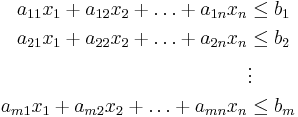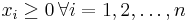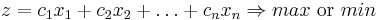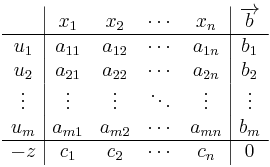# Informatics1-2017/Practice7

## Latex mathematical formulas

### Formulas

Reproduce the following in latex:\begin{align} a_{11}x_1 + a_{12}x_2 + \ldots + a_{1n}x_n &\leq b_1\\ a_{21}x_1 + a_{22}x_2 + \ldots + a_{2n}x_n &\leq b_2\\ &\vdots\\ a_{m1}x_1 + a_{m2}x_2 + \ldots + a_{mn}x_n &\leq b_m \end{align}

where$x_i \geq 0 \, \forall i=1,2,\ldots,n$. The form of the objective function:$z = c_1x_1 + c_2x_2 + \ldots + c_nx_n \Rightarrow \mathop{max} \text{ or } \mathop{min}$

The same with vectors and matrices:\begin{align} \overrightarrow{x} &\geq 0\\ A\overrightarrow{x} &\leq \overrightarrow{b}\\ z=\overrightarrow{c}^T\overrightarrow{x}&\Rightarrow\mathop{max} \text{ or } \mathop{min} \end{align}

### Matrices, tables

Try the tabular environment with different aligns! Create a 3x3 matrix with all kinds of brackets. Try to do an nxn matrix as well for example, try to create this formula from wikipedia: matrix.

Reproduce this table (matrix):$\begin{array}{c|cccc|c} & x_1 & x_2 & \cdots & x_n & \overrightarrow{b}\\\hline u_1 & a_{11} & a_{12} & \cdots & a_{1n} & b_1\\ u_2 & a_{21} & a_{22} & \cdots & a_{2n} & b_2\\ \vdots & \vdots & \vdots & \ddots & \vdots & \vdots\\ u_m & a_{m1} & a_{m2} & \cdots & a_{mn} & b_m\\\hline -z & c_1 & c_2 & \cdots & c_n & 0 \end{array}$

### Theorems, definitions

Let's do some theorems. For that append this to the preamble:

\newtheorem{mydef}{Definition}
• Create a new threorem style environment!
• Try the different styles (remark, theorem, definition)!

### Labels, references

\begin{theorem}\label{thm:sample_thm}
Theorem text
\end{theorem}

In Theorem \ref{thm:sample_thm} we...

#### Floating pictures

\begin{figure}[p]
\centering
\includegraphics[width=0.8\textwidth]{image.png}
\caption{Awesome Image}
\label{fig:awesome_image}
\end{figure}

Change the placement (h,t,p,b,!,H)!

## BibTeX

BibTeX is a package to create nice looking bibliographies. Create a test bibliography using these sites: http://www.bibtex.org/Using/, https://en.wikibooks.org/wiki/LaTeX/Bibliography_Management.